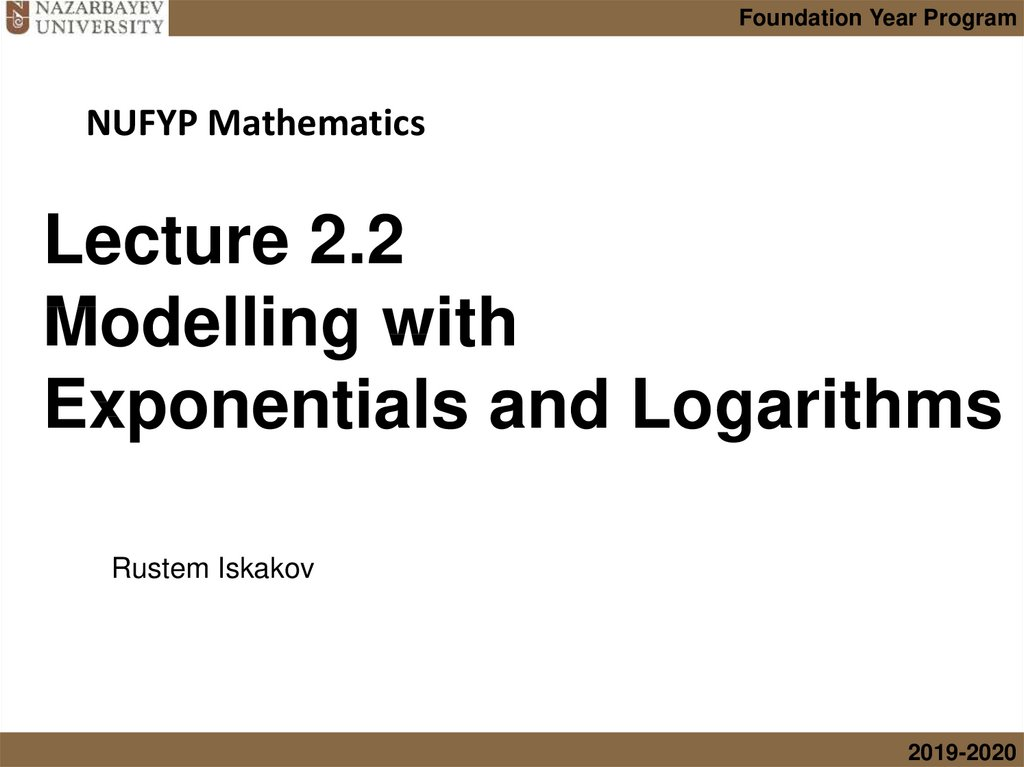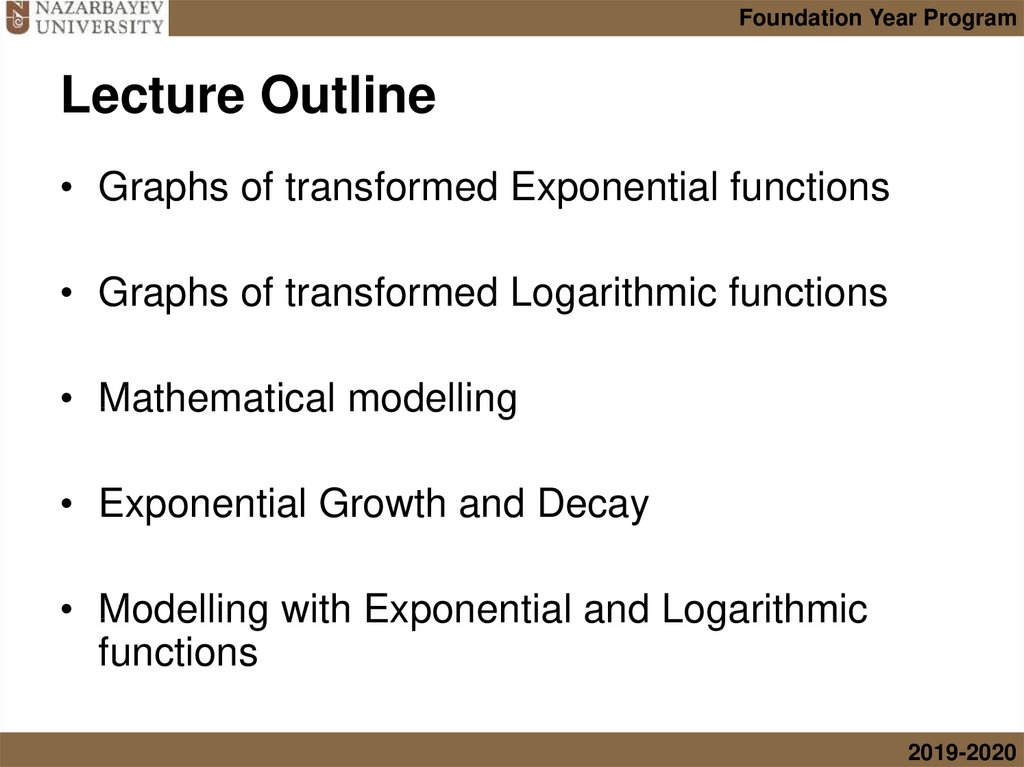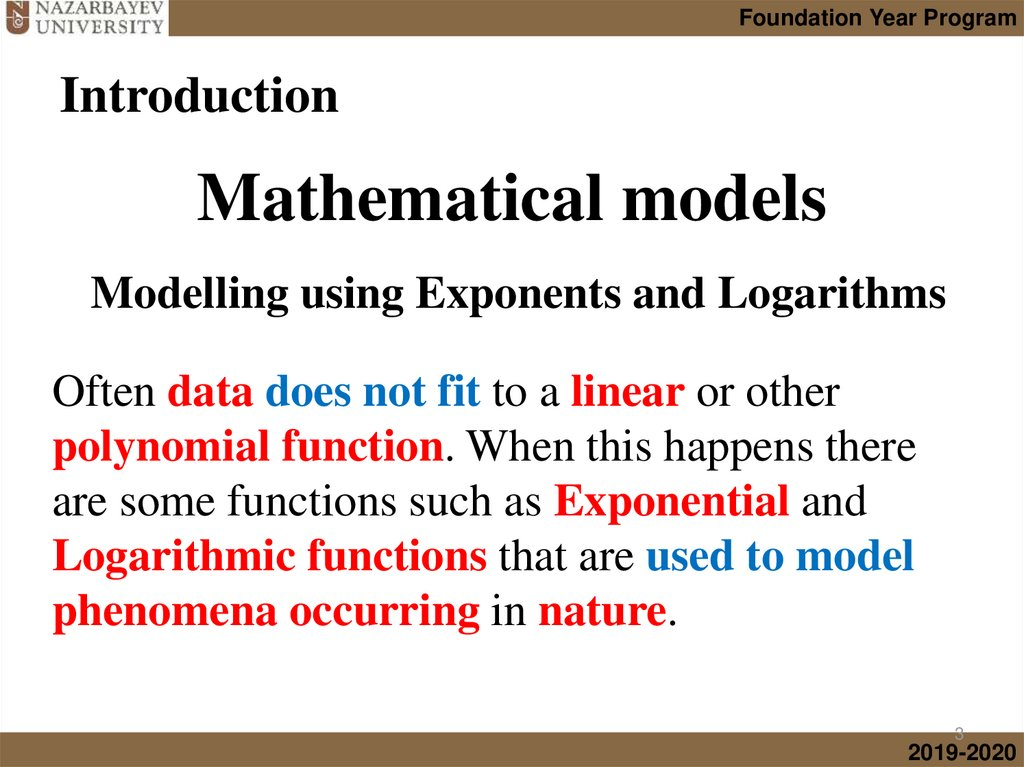# Modelling with Exponentials and Logarithms

## 1. Lecture 2.2 Modelling with Exponentials and Logarithms

Foundation Year Program
NUFYP Mathematics
Lecture 2.2
Modelling with
Exponentials and Logarithms
Rustem Iskakov
2019-2020

## 2. Lecture Outline

Foundation Year Program
Lecture Outline
• Graphs of transformed Exponential functions
• Graphs of transformed Logarithmic functions
• Mathematical modelling
• Exponential Growth and Decay
• Modelling with Exponential and Logarithmic
functions
2019-2020

## 3. Introduction

Foundation Year Program
Introduction
Mathematical models
Modelling using Exponents and Logarithms
Often data does not fit to a linear or other
polynomial function. When this happens there
are some functions such as Exponential and
Logarithmic functions that are used to model
phenomena occurring in nature.
3
2019-2020

## 4.

Foundation Year Program
Mathematical models
1. Exponential Growth
Used to model:
• Population growth
• Compound interest
2. Exponential Decay
Used to model:
• Carbon dating
4
2019-2020

## 5.

Foundation Year Program
Mathematical models
3. Logarithmic Growth
Used to model:
• Earthquakes
• Sound levels
4. Logistic Growth
Used to model:
• Learning
5
2019-2020

## 6.

Foundation Year Program
Mathematical models
5.
Gaussian distribution (Normal distrib.)
Equation:
Used to model:
• Probability
distribution
• Standardized
test (SAT)
marks
6
2019-2020

## 7.

Foundation Year Program
Mathematical models
1. Exponential Growth
2. Exponential Decay
3. Logarithmic Model
Note: In this
lecture we will
focus only on
these 3 models
4. Logistic Growth
5. Gaussian Distribution (Normal Distribution)
7
2019-2020

## 8.

Foundation Year Program
Introduction to “e”
Mathematical constant e is a real, irrational and
transcendental number approximately equal to:
e = 2.71828 18284 59045 23536 02874 71352 66249 77572 47093 6999595749
66967 62772 40766 30353 54759 45713 82178 53516 6427427466 39193 20030
59921 81741 35966 29043 57290 03342 9526059563 07381 32328 62794 34907
63233 82988 07531 95251 01901 …
A transcendental number is a number that is not a root of any
polynomial with integer coefficients. They are the opposite of algebraic
numbers, which are numbers that are roots of some integer polynomial.
Do you know any other transcendental number?